The timeline spiral.
Let us take a point as begining of everything in the example. Place existed of this point and time is place of limit approached from negative to 0. Let us take minimal quantity of passage of time conditionally (T). If we accept to take the know point all matter or the universe then they received the following situation: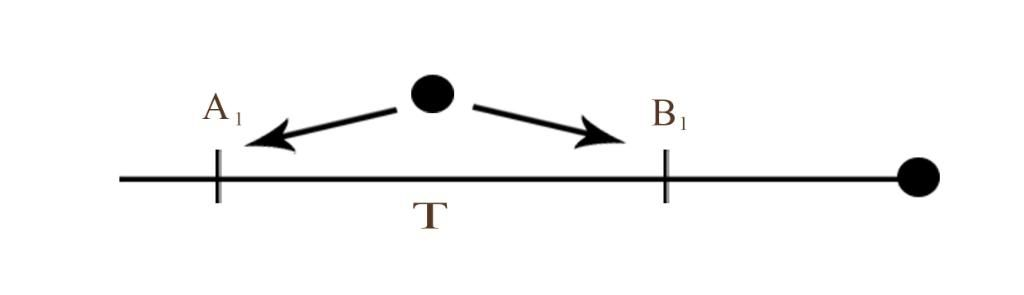Then for the next past (T) time: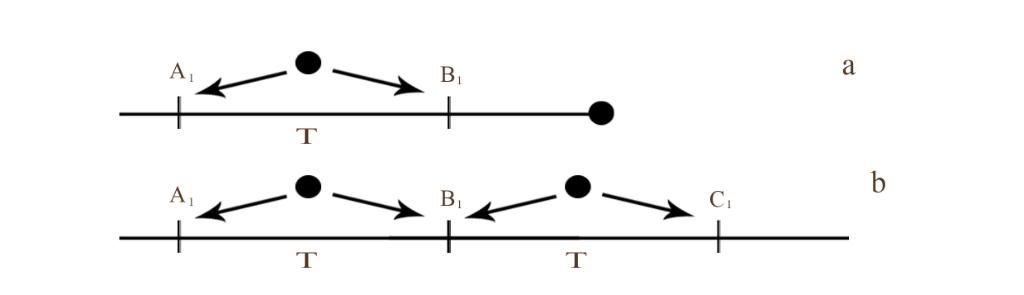Here a and b is the new timeline arising when each (T) time is passed. If we include the conception of paralel universes here, then will arise of the following shaped spiral lines: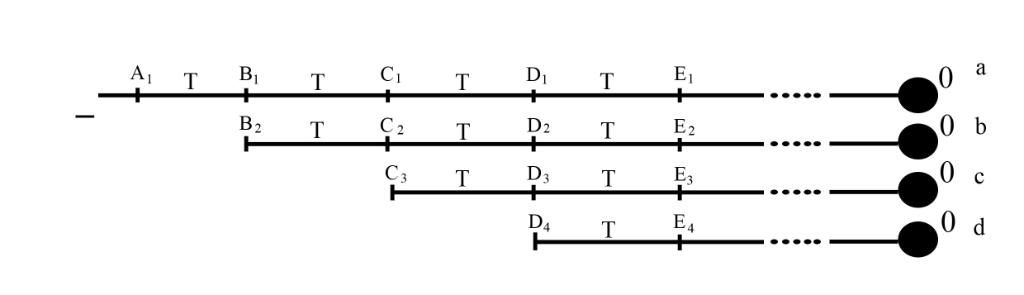Here: a, b, c, d are the lines of the time spiral.
If we imagine that there is thing N1 in our hand. Then this N1 thing is created from the the thing N2 during the (T=a) time. N2 was created from the N3 during (T=b) time and etc. If we conditional that non-existence arises onle from the non-existence, the nothing does not arise from the non-existence. Then source of the N1 goes it turns out the a, b, c and etc. cuts of time true to the negative and it is endless. Then that time lines of the spiral to the left side lies to the negative endlessness
In the physics and astronomy there is a conception about the black hole where the matter and time disappears. If we imagine that matter dissapears in the black hole then zero point of the universe will not it`s begining . It will be universeses end point. So here we shall take our beginning from the endless to the negative to the left side of timeline spiral, and end like the 0 point to the right side of the timeline spiral. Then the lines of the spiral lies to the 0 to the right side. And rises the following forms of spiral: (This is the 2D view of the timeline spiral.)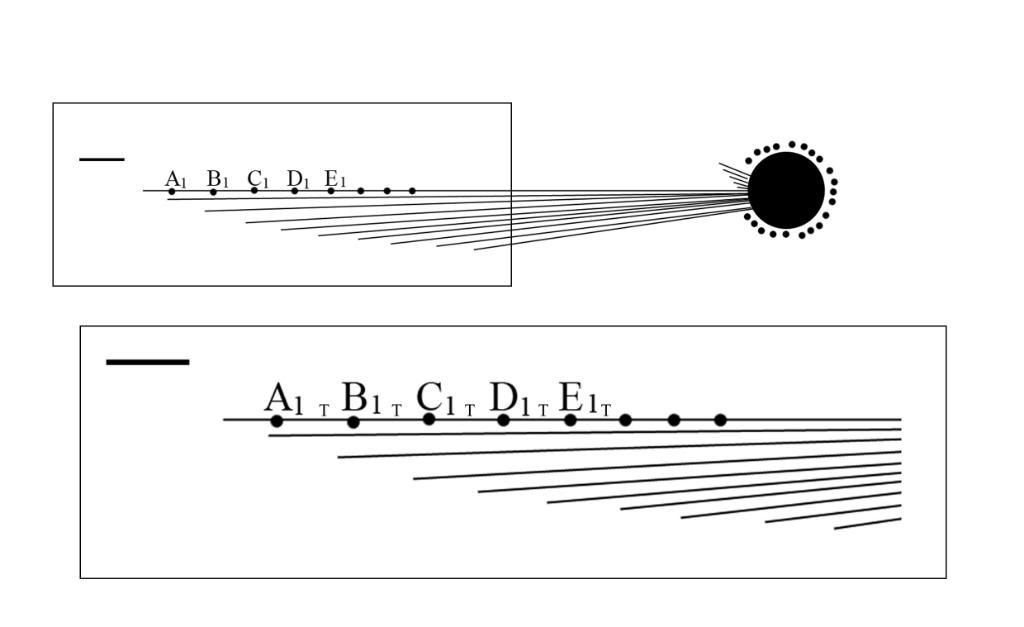Go to the forward and motion back in the spiral of timeline.
Motion back ( Go to past)
How faster will go the thing so slowly will go time inside it. If we imagine that the speed of the thing is (S), then the speed of time inside it will be (ST). Then if the speed of the thing will increase x and become (xS), speed of the time inside will be (ST/x). Speed of the thing is stubborn proportionate with the speed of time inside the thing. If we look against we can come such conclusion that how slower thing will go so faster will go time inside it. Minimum motion speed is 0. We can subconsciousness to get negative speed and go forward unreal or advance the possible preiod of in our thought if we only get up in the peace imaginary.
Let us take (A1) in the example of the thing. after the (t1) time the thing is changes and becomes (B1), after the (t2) time it becomes (C1) like the same way and etc. In the timeline spiral it reflectes like so: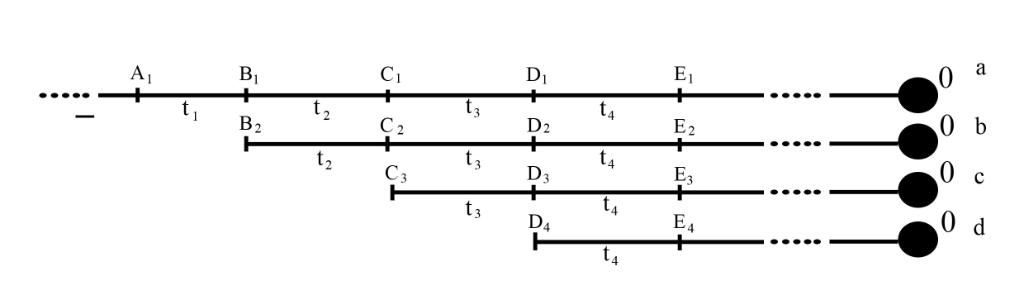Now the matter begins from the negative and move along the timeline and after (tn) time a new timelines begins with the (An), (Bn+1), (Cn+2) and everything is happening the same parallely along this lines. So (B1) = (B2) ; (C1) = (C2) = (C3) (or against).
Now we got a question, the thing which is on (A1) moves to the (B1), after that it moves to the (C1). And if it is possible motion back, and when we go past from (C1) to (B2) and change something there, then does the (B2) moves to (C2) different from, (B1) moves to (C1)? Then how the (C1) = (C2).... low will protected?
If we move from (C1) to (B2) and make some changes on (B2) it may happen in the following forms:
In the first form: If the spiralic space is to much bigger between (C1) and (B2), then after the changes on (B2) difference between (C2) and (C1) approaches to 0. Volume of the approaching is stubborn proportionate with the volume of the spiralic space.. (How bigger will the volume of the spirallic space difference will be so nearly to 0 so it will be so smaller.) It can also become in the multidimensional timeline spiral. It may also become against. So, how smaller will the volume of the spirallic space differense will be so nearly to 0. We only intended the slackenging time of the period in this form.
For example if we move to the time where lived a dinosaur and broke there a leaf it will not change our todays with a great difference, that it means that the difference is nearly to the 0. Or if we sit in the time machine and after experiment we test our watches and we see that our watches is stayed behind 1 or 2 minutes it is also will not change our todays with a great difference. And it just only means we slackened the time.
In the second form if we move back from (C1) to (B2), then the changes which we did on (B2) will continue till we move to (C2) or some other point of the timeline untill we was there and when came back to (C1) we see that the changes was lost.
For example we go to past and see that we are in the center of the war. And we stay there 2 minutes. In this 2 minutes somebody kills us. When the time machine call us back we began to live the back all what happened. And when the two minutes comes to the 0 in the time where we reached that place then we began to come our days. We shall call this two minutes (t0). This (t0) goes to the spirallic space so, the people don`t feel that who was in that war. (t0) is the part of the timeline which is broken from the timeline and concerns to the spirallic space.
In the third form we know that, (B1) = (B2) ; (C1) = (C2) = (C3) (or against) then, (B2) = (B1) ; (C3) = (C2) = (C1) (or against). So if we move from (C1) to (B2) and make some changes on (B2) that (B2) becomes (B2)` then (B2)` = (B1)`.So when we come back we shall be in the (C1) which is reachenged by (B2) we shall be in the (C1 ) it can only be possible when the changes on (B2) borns (B2)`, in the situation on (C1)` let him to return (C1.) If it is prevent to return the thing which make a changes dissapears ot concerns to spirallic space and goes to the endlessness. So it is a variant of the appearance. That we see but not interfere physicly.
Let us do the conditional situations additions over the straight line of the timeline:
Motion back
(In every situation (T1) is the time when the thing began moved to the past, (T2) the time when the thing come back from the past, (T3) the time where the thing reached the past, (T4) when the thing begin return from the past, (t1) time which spelling on the way to the past, (t2) time which spelling in the way from the past).

I. If
(T2) - (T1) is near or equal to 0
(T4) - (T3) > 0 and (T4) - (T3) > (T2) - (T1)
(t2) + (t1) is near or equal to 0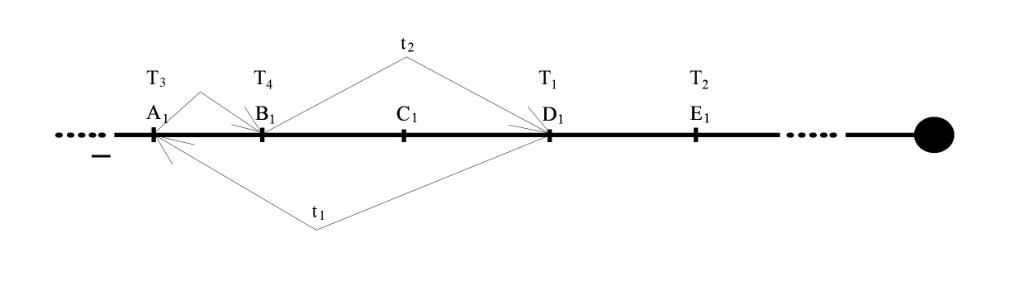The thing in the (T2) - (T1) wastes or falls behind the time such ((T4) - (T3)) + ((t1)+ (t2))

II. If
(T2) - (T1) is near or equal to 0
(T4) - (T3) is near or equal to 0
(t2) + (t1) is near or equal to 0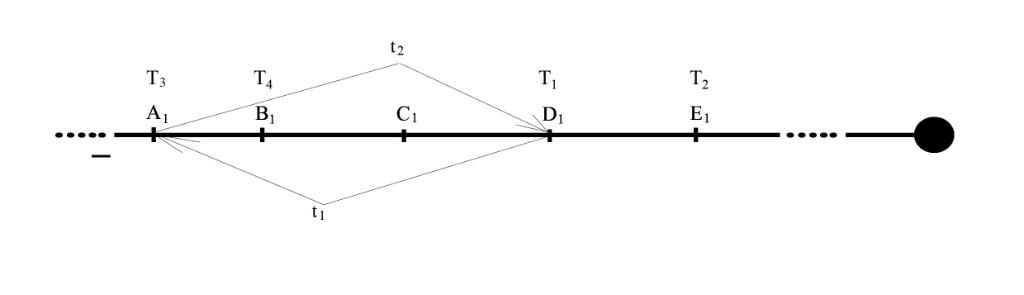It is the rising of the timeline spiral accortding to the past time.

III. If
(T2) - (T1) > 0
(T4) - (T3) is near or equal to 0
(t2) + (t1) is near or equal to 0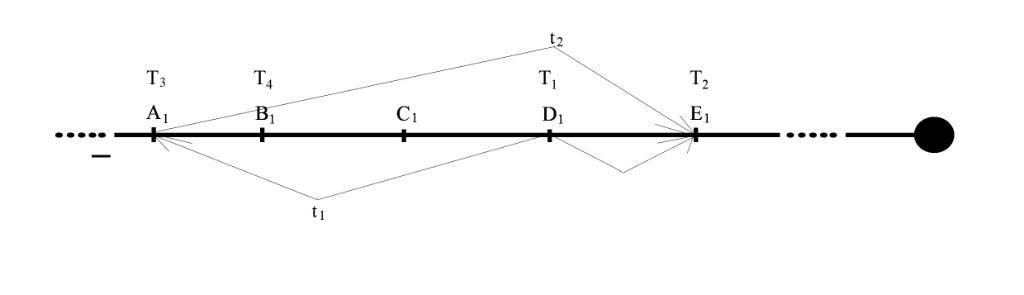The thing in the time ((T4) - (T3)) + ((t1)+ (t2)) earns or falls forward the time such (T2) - (T1)

IV. If
(T2) - (T1) > 0
(T4) - (T3) > 0
(t2) + (t1) is near or equal to 0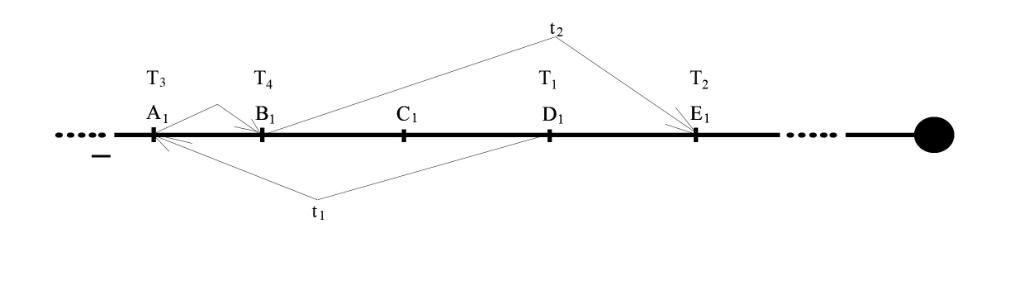And if the (T2) - (T1) = ((T4) - (T3)) + ((t1)+ (t2)) then nothing changes with the time of the thing.

V. If
(T2) - (T1)=0
(T4) - (T3)=0
(t2) + (t1)<0It is the rising of the black hole effect according to the past.

VI. If
(T2) - (T1)=0
(T4) - (T3)=0
(t2) + (t1) >0In the 0 time thing waste or falls back time such (t2) + (t1).

VII. If
(T2) - (T1)=0
(T4) - (T3)=0
(t2) + (t1) =0It is the constance situation of the thing.

The trajectory which the thing moves from (D1) to (A1) is measured with definite imaginary distance which is shown on the spirallic space with a time. So (t1) and (t2) is so conceptions that we can not feel and count them because of they happens in another universes. If we imagine that the time difference between (D1) and (A1) is (T) and the line distance between them is (D1A1).
Then (t1)= (D1A1)/ (T) and if we take it like we feel then (t1) < (T) But it really must be against. So it must be (t1) > (T). Here we have straight proportionality. So how bigger will the (t1) so longer will be (D1A1). It means that how longer the thing will on the to the past so past it will get.

Motion forward ( go to future)

Let us take an example under the meaning the rising timeline spiral according to the past, if the man goes to the past (00:00) in the 00:01 and instandly returns from the 00:00 and see that the time is 00:01 after 1 second which he does not feel. He didn`t feel anything he sees the time 00:00 then 00:01.Before we explained that we can not feel this travel time and distance. Then it means that when we are in the 00:00 in reality we are in the 1 second before 00:01.If we imagine the 1 second conditional then it can also be with a great difference. It gives the theoretical base of the forming timeline spiral according to the future. And maybe explains how to know in 00:00 what will happen after 1 second in 00:01 which we call dejaview.

Let us explain possibility of the going to the future in the condition of the existing of the timeline spiral according to the future. According to the general form if we have timeline spiral then we move from the past to the future towards 0. But because of all the lines of spiral are interseced in 0 then if take against it means that they also begining in the 0. So all the life is existed from 0 to negative than from the end to the beginning we live and move staright from the beginning to the end. It means that if we are in the 2009 the reality it that we are in 1 year past of the happening 2010. The 2010 is already existed in the timeline spiral.
Let us take some example. Imagine that we are in the 2009. And we move to the future in the year 2050. And there we see a technology which we don`t know in 2009. We learn everything about this technology and return to the 2009. It means that also when we reach to the 2050 some people from the 2009 will come and learn from us that technology. And in the 2050 where we learned about the technology there the people also in there life in 2009 went to the 2050 and get that texhnology.
Here we got questions. When this technology created?
If we take example real it goes to the endlessness and goes to the negative like the beginning of the timeline spiral. If some one goes to the future and learns a new technology and apply it in his time, then on the timeline between the 2 points cuting and losing a time in form of like (t0) which is includes to the spirallic space. The technology was made in the (t0). And we don`t feel it.
If we generalize all of what we said we get such though: We are in the (A1) because there will be (B1) and (B2) but according to the theory of timeline spirall it has already was. we are in the (B1) or (B2) because there will be (C1), (C2) or (C3) but they has already was. If go against (C1), (C2) or (C3) is because (B1) or (B2) was, (B1) or (B2) is because (A1) was. How become (A1) ? If we take into account that anything does not arise from non-existence. Before (A1) was non-existence, then non-existence was because (A1) will or already was.
Let us do the conditional situations additions over the straight line of the timeline:

Motion forward
(Here (T1) the time when we began go to the future, (T2) time when we reach back from the future, (T3) the time we we reach the future, (T4) when we return from the future, (t1) time which is spelling on the way to the future, (t2) time which is spelling on the way from the future).

I. If
(T2) - (T1) is near or equal to 0
(T4) - (T3) is near or equal to 0
(t2) + (t1) > 0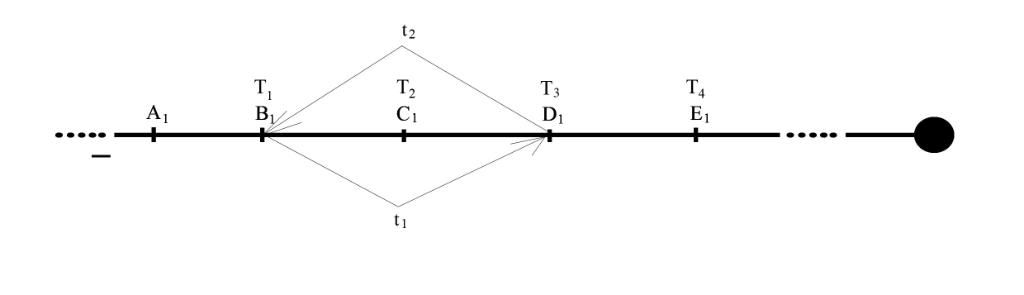The thing in the (T2) - (T1) wastes or falls behind the time such (t1)+ (t2)

II. If
(T2) - (T1) is near or equal to 0
(T4) - (T3) > 0
(t2) + (t1) is near or equal to 0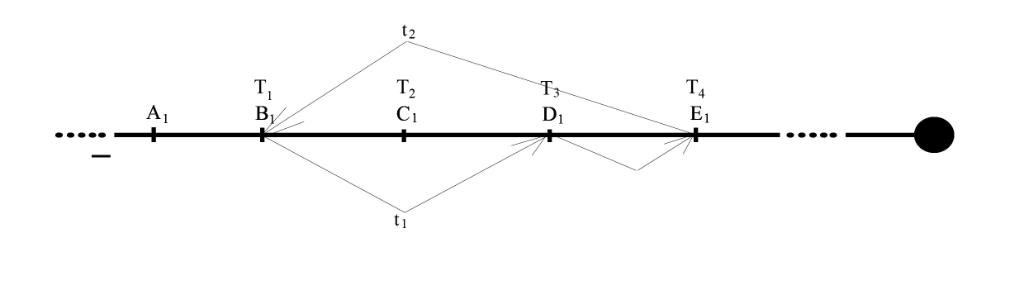The thing in the (T2) - (T1) wastes or falls behind the time such ((T4) - (T3)) + ((t1)+ (t2))

III. If
(T2) - (T1) > 0
(T4) - (T3) is near or equal to 0
(t2) + (t1) > 0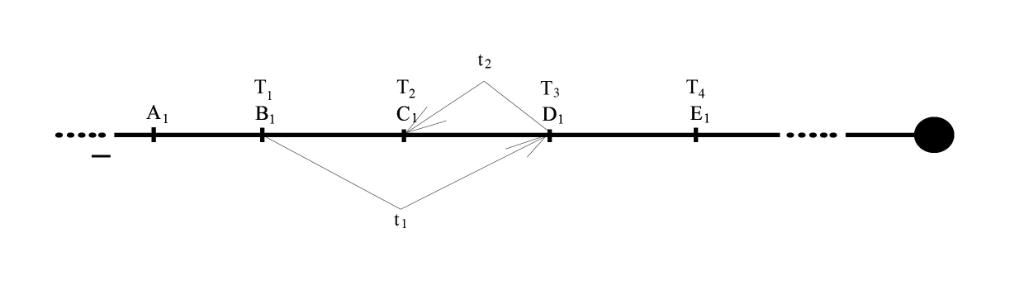The thing in the time (t1)+ (t2) earns or falls forward the time such (T2) - (T1)

IV. If
(T2) - (T1) > 0
(T4) - (T3) > 0
(t2) + (t1) =0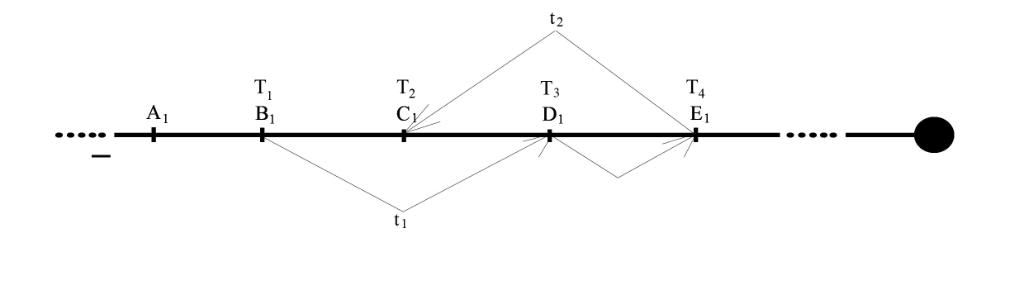Nothing changes about the time for the thing
if the (t2) + (t1) > 0 The thing in the (T2) - (T1) wastes or falls behind the time such (t1)+ (t2)

V. If
(T2) - (T1) is near or equal to 0
(T4) - (T3) is near or equal to 0
(t2) + (t1) is near or equal to 0It is a rising of the timeline spiral according to the future.
By the same conditions if (t2) + (t1) < 0 it is the rising of the black hole effect according to the future.

VI. If
(T2) - (T1) =0
(T4) - (T3) =0
(t2) + (t1) =0It is the constance situation of the thing.

General situation:
We can motion back:
1. If we dont influence physically and just only in the case of view.
2. Go to the to very past which any changes over there will not actually change our todays.
3. Under the conditions of the conceptions of cut of tense (t0).

We can motion forward:
1. If we dont influence physically and just only in the case of view.
2. Under the conditions of the conceptions of cut of tense (t0).

If the period of the motion between the parallel lines of the timeline spiral is (tn)<0 it is the effect of the black hole.
Because of the parallel lines of the timeline spiral joins on the 0 point, they are intersected.
The distance, time and other measures between the parallel lines of the timeline spiral creates the spirallic space.
Spirallic space is made from (t0). (t0) includes (or comes from timeline).
The timeline spiral is much dimenesions, unreal and goes from the 0 to the negative endlessness and against.
The time moves on the timeline spiral against. We live it straight.
The timeline spiral iz imaginary.
The same named and different numbered points on the parallel lines of the timeline spiral are equal.
Events in the sleep of man happens in the spirallic space.

(Explanation of the paranormal events in the timeline spiral) - we can explane all the paranormal events which was happend in the time case.